﻿ Proof the Riemann Hypothesis

### Proof the Riemann Hypothesis

P.M. MazurkinOPEN ACCESSPEER-REVIEWED

## Proof the Riemann Hypothesis

Doctor of Engineering Science, Academician of RANS, member of EANS, Volga Region State Technological University, Russia

### Abstract

In the proof of the correctness of the Riemann hypothesis held strong Godel's incompleteness theorem. In keeping with the ideas of Poja and Hadamard's mathematical inventions, we decided to go beyond the modern achievements of the Gauss law of prime numbers and Riemann transformations in the complex numbers, knowing that at equipotent prime natural numbers will be sufficient mathematical transformations in real numbers. In simple numbers on the top left corner of the incidence matrix blocks are of the frame. When they move, a jump of the prime rate. Capacity of a number of prime numbers can be controlled by a frame, and they will be more reliable digits. In the column i=1 there is only one non-trivial zero on j=(0,∞). By the implicit Gaussian normal distribution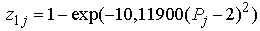, where Pj - a number of prime numbers with the order-rank j. On the critical line of the formula for prime numbers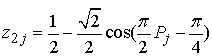. By the famous Riemann hypothesis is that the real part of the root is always exactly equal to 1/2 is obtained - the vibration frequency of a series of prime numbers is equal π/2, and the shift of the wave - π/4.

### At a glance: Figures

1234
Prev Next

• Mazurkin, P.M.. "Proof the Riemann Hypothesis." American Journal of Applied Mathematics and Statistics 2.2 (2014): 53-59.
• Mazurkin, P. (2014). Proof the Riemann Hypothesis. American Journal of Applied Mathematics and Statistics, 2(2), 53-59.
• Mazurkin, P.M.. "Proof the Riemann Hypothesis." American Journal of Applied Mathematics and Statistics 2, no. 2 (2014): 53-59.

 Import into BibTeX Import into EndNote Import into RefMan Import into RefWorks

### 1. Introduction

While working on the proof of the correctness of the Riemann hypothesis held strong Godel's incompleteness theorem: "The logical completeness (or incompleteness) of any system of axioms cannot be proved within this system. For its proof or refutation of the required additional axioms (strengthening of the system)".

The incompleteness of the known law of distribution of prime numbers is as follows:

1) in order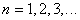not considered zero (truncated natural numbers);

2) a traditional series of prime numbers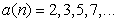does not include zero and one ;

3) the assumption that "the ratio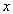tothe transition from a given degree of ten to follow all the time increases by about 2,3" is clearly incorrect ;

4) a statement that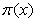~, proposed in 1896 by Gauss, takes the prime numbers from the decimal number system in a number system with base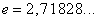;

5) the power of prime numbersin a series of natural numbersfrom the orders taken in decimal arithmetic, and the ratio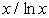- in base of natural logarithms.

In keeping with the ideas of mathematicians Poya and Hadamar about mathematical inventions, we decided to go beyond the modern achievements of the Gauss law of prime numbers and Riemann transformations in the complex numbers, realizing that at equipotent prime natural numbers will be sufficient mathematical transformations in real numbers .

### 2. Full Range, Methods and Data

Prime number - this is a natural number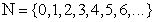with a natural divider 1 (division by himself – is redundantly).

Of prime numbers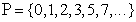"ladder of Gauss-Riemann" separated "step" increase with the parameter of prime numbers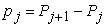, where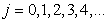- is the rank order. Rejection of the system with baseled to the translation of the binary system. Understand that mathematics, fascinated by the factorization of prime numbers, forget about the benefits of the decomposition of numbers.

Table 1 shows the conversion of 500 prime numbers from decimal to binary. The decomposition of primes is known by the simple rules on the discharge rank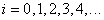. Then, a binary (real) numbers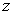were revealed distinctive features of the distribution of prime numbers and their growth on critical lines of the Riemann and envelope of the left border lines 1.

Factor analysis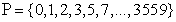gave the number 1/2.

#### Table 1. Parameters of the total number of 500 prime numbers in binary

Table 1 shows the symmetrical geometric patterns, but their analysis we did not. It is seen that any prime number before itself has a ratio of 1/2. But it is a sum of terms is not included. Complex mathematical expressions, the parameters of the series has the form:(1)(2)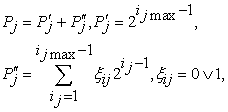(3)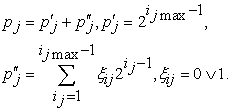(4)

In Table 1 we have two types of zeros - the trivial (empty cells) and unconventional (0). The first prime numbers to the left of the broken line 1. A non-trivial zeros lie inside the two columns 1. More complicated with the growth - the bending around all j1 is the wave, and the line itself is always concerned the critical line.

### 3. Mathematical “landscape”

In the film «De Code» (19.07; 26.07 and 02.08.2011) showed a three-dimensional picture of the Riemann zeta function. All pay attention to the nontrivial zeros on the critical line. They are already counted several trillion.

Alignment of the binary system is infinitely high "mountain" transforms into ledges of identical height, equal to unity. Figure 1 shows the landscape of the 24 first prime numbers.

Figure 1. Mathematical “landscape” binary distribution of the 24 first prime numbers

In Figure 1, there is a "ceiling" of 1, except the "floor" of the nontrivial zeros. Between them there is an unknown factorial relationship. Then the hard surface of the Riemann zeta function, due to the submission of complex numbers is transformed into "two-layer cake."

### 4. Benchmarks

They are on the upper left corner blocks of prime numbers. It was during the transition to them occurs a jump increase in prime. Therefore, power series of prime numbers is quite possible to manage with the help of a benchmarks, they will be safer decimal digits.

From Table 1, we write the nodal values(Table 2) and other parameters of benchmarks.

#### Table 2. Asymptotic benchmarks a number of 500 primes

Power measurement range of prime numbers on benchmark is much more economical.

### 5. Influence of Prime Numbers Per Grade

Table 1 shows, atthere is.

And in the column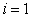(Figure 2) there is only one nontrivial zero throughout, i.e. before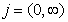.

By implicitly [3, 4] given us the law of Gauss "normal" distribution have(5)

Then the prime number 2 is a critical and noncritical series begins with 3.

On the critical line is the formula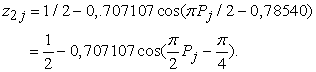(6)

Completed (Figure 3) evidence of "the famous Riemann hypothesis about that the real part of the root is always exactly equal to 1/2". The frequency of oscillation is equal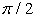, and the shift -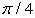.

There were two fundamental physical constants: in formula (5) - Napier number(the number of times) in equation (6) - Archimedes number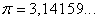(the number of spaces). What does it mean 0,707107 - we do not know.

Then obtained (Figure 4) model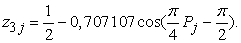(7)

Montgomery and Dyson applied statistical physical methods of the analysis of distributions with respect to a number of primes and determined the average frequency of occurrences of zeros.

But it turns out, the average frequency over the binary conversion of prime numbers is obtained by functionally related to the number of spaces.

From the remains of up to 0,25 for the fourth level was obtained (Figure 4) model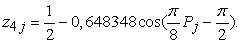(8)

For the fifth and sixth digits (Figure 5) were obtained regularities: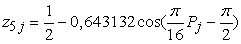(9)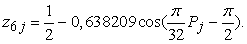(10)

It is noticeable that with increasing level binary system balances (absolute error) increases. This can be seen in the graphs to reduce the correlation coefficient.

In 1972 proved nature of the distribution of the zeros on the critical line. From formulas (6) and other shows that they (and 1) is indeed fluctuate. We explain the desire of prime numbers, as well as convert them to binary 0 and 1, diverge from each other because of the power produced in the progression. A nontrivial zeros of scatter in the plane (,) in laws (3) for summandat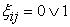.

For the seventh and eighth categories (Figure 6) formulas of a similar design are received:(11)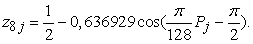(12)

For the ninth and tenth digits (Figure 7) have produced similar pattern: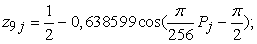(13)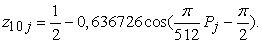(14)

For the 11-th digit (Figure 8) similarly has been received the formula (with the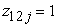)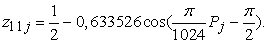(15)

There are among mathematicians claim: in the distribution of prime numbers there is no geometry. The pattern on the binary matrix in Table 1 and for residues in Figure 8 disprove this statement. However, what and how to identify them, we do not yet know.

### 6. Effect of Growth in Charges

Bernhard Riemann in 1859, according to the analysis of the zeta function asserted that the zeros are on the same line. Now believe it as critical line crosses the mathematical landscape of the zeta function.

From the data of Table 1 shows that for the new parameter of a number - increase of the number of primes - there is a single line. This - the vertical. We show that the rest of the vertical, of the components of the primes slowly approaching the critical line on the condition.Thus once again confirming the proof of the Hardy about the fact that in a number of there are an infinite number of non-trivial zeros, part of which can not lie on the critical line.

For 1 and 2 categories (Figure 9) on unbroken trivial zeros of the verticals are: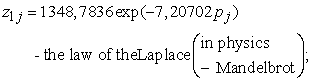(16)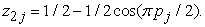(17)

The critical linehas received the unequivocal formula, and without wave shift.

In our example, from 500 in increments of prime numbers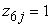. For 3, 4, and 5 bits are excluded from the vertical cell with trivial zeros.

The general equation for these lines receives a variable frequency of the oscillation. For 3 or 4 digits (Figure 10) were obtained by the formula: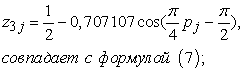(18)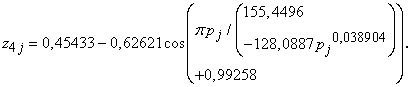(19)

Even greater deviation from the critical line (Figure 11) is on the fifth category:(20)

### 7. The Beginning of a Critical Line

Formula (17) is observed at the minimum short of the critical line containing two points (Figure 12).

### 8. Conclusions

The famous Riemann hypothesis is proved. For this was accomplished the transformation of a number of prime numbers from decimal notation to binary. We obtain four new criteria. There were geometric patterns. Became visible "on the floor" non-trivial zeros and appeared units "on the ceiling" of the distribution of 0 and 1 instead of abrupt "hills" of zeta-function.

### References

  Don Zagier. The first 50 million prime numbers. URL: http://www.ega-math.narod.ru/Liv/Zagier.htm.In article  Number. URL: http://ru.wikipedia.org/wiki/%D0%A7%D0%B8%D1%81%D0%BB%D0%BE.In article  Mazurkin P.M. Biotechnical principle and sustainable laws of distribution // Successes of modern natural sciences. 2009. № 9, 93-97.In article  Mazurkin PM The statistical model of the periodic system of chemical elements D.I. Mendeleev. Yoshkar-Ola: MarSTU, 2006. 152.In article